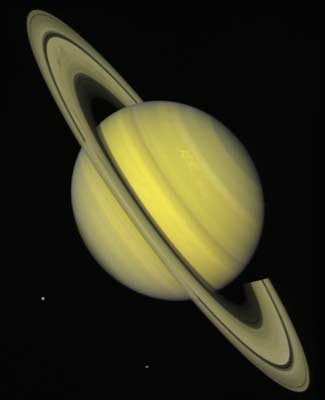The Easy Solution
to the New Saturn Anomaly

by Miles MathisIn November of 2008, Lorenzo Iorio published an announcement with ArXiv1 that E.V. Pitjeva had analyzed Cassini spacecraft data, finding that the precession of Saturn did not conform to the predictions of GR. The gap between data and prediction was calculated to be .004-.008 arcsec/cy. The announcement in ArXiv corresponded to a paper by Iorio and Ruggiero in SRX Physics2 at about the same time, in which the authors showed that theories of long range modified gravity (LRMG) could not explain the gap. This was in preparation for a paper by Iorio in July, 20093, which proposed that an undiscovered planet X was causing the gap.

This is a drôle repeat of history, since in 1859 Le Verrier proposed the planet Vulcan as the solution to the original precession problem with Mercury. But we need neither a new planet X nor any LRMG. The only "modification" we need to gravity is the simple one I have shown in my long paper on the precession of Mercury. We don't need to "modify" gravity at all: we only need to correct the simple mistakes in the field equations we have. In fact, my finding that Einstein's field equations are 4% wrong in the field of the Sun is enough by itself to solve the Saturn anomaly. The accepted value for the precession of Saturn is .1836 arcsec/cy.4 If we multiply that by .04, we obtain .007. That is nearly in the middle of the range calculated by Pitjeva, as you see. I solved the problem before I even knew of it. To put it another way, I predicted in 2007 that Einstein's field equations were 4% wrong. In 2008, that prediction was confirmed by data from Saturn.

I will repeat the short math I did in the other paper. Einstein's field equations are field equations of mass, as everyone should know. To solve, Einstein basically does a mass transform in a curved field. In other words, he takes his mass transform from SR and imports it into his tensor equations. The problem with this is that gravity is not a mass, it is a force caused by an acceleration field. Einstein needs to transform a force, but he only transforms a mass. Since by the classical force equation F = ma, force is measured in Newtons (kilogram meter per second squared), Einstein needs to transform mass, length, and time squared all in the same equation. I showed that in a given event, Mercury's mass would increase 1.57 times, while its length would increase by 1.04 and its time would decrease by 1.04. Since F = ma, our aggregate transform is just F = (1.57)(1.04)/(1.04)2 = 1.51. The difference between 1.57 and 1.51 is 4%. Since Einstein is only transforming mass, his field equations must be 4% wrong across the board.

Critics will now say that my solution is just another LRMG, or a modified gravity, but it should be clear that it is not. One, because it is not an external modification to GR. It is simply a correction of an internal mistake. Two, because it is not an ad hoc addition to the field. I just showed you why my correction works, and none of the other LRMG's can do that. All the other "theories" are just gap fillers, created to answer specific shortcomings. My mathematical correction is a general solution, complete with all the mechanics. Nor does my solution fall to Iorio's SRX Physics paper, since my solution, although general, has to be applied to each known precession separately. My 4% correction applies to each problem, not to sets of problems. To be specific, Iorio and Ruggiero do the math for the perturbations between sets of planets, as in ΨJupSat = 1.36 ± .06. They find different values for different planet pairs, and conclude that LRMG's can't explain this variance. It is true that LRMG's can't explain this, but I can. Although perturbations cause precessions, calculating perturbations and calculating a change in precession on a single planet are two different things. In finding the number .04, you can see that I am calculating a general correction to the field. To apply that general correction to a specific perturbation between given planets requires more math. Specifically, it requires taking into account the two masses and the distance between them. Because the distances and masses are not equal, we should not expect the field errors or corrections to be equal. Iorio and Ruggiero's equations rule out the logarithmic correction and the power-law correction to gravity, but don't rule out my correction. In fact, their equations confirm my correction, since their tables 13 and 21 show that the variance in perturbations is a factor of distance and mass.

For more confirmation of my 4% correction to GR, we may look at The 1983 experiment of Bonse and Wroblewski,11 in which they used neutron interferometry and oscillation to nearly cancel interfering beams. They found that gravitational effects were equivalent to acceleration effects in such a measurement, to within 4%. This 4% error has never been explained until now, but it is clear at a glance that my math aboves solves it. Bonse and Wroblewski used the current GR equations to calculate the equivalence of acceleration and gravity, and those equations were simply wrong by 4%.

1http://arxiv.org/abs/0811.0756
2http://www.syrexe.com/physics/2008/968393.html
3http://arxiv.org/abs/0907.4514
4http://farside.ph.utexas.edu/teaching/336k/lectures/node128.html

If this paper was useful to you in any way, please consider donating a dollar (or more) to the SAVE THE ARTISTS FOUNDATION. This will allow me to continue writing these "unpublishable" things. Don't be confused by paying Melisa Smith--that is just one of my many noms de plume. If you are a Paypal user, there is no fee; so it might be worth your while to become one. Otherwise they will rob us 33 cents for each transaction.